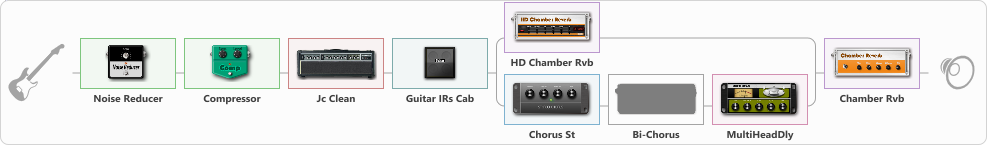# Moon clean

Discussion in 'ToneLib-GFX presets' started by truss van halen, Dec 17, 2020.

1. ### truss van halenWell-Known Member

Moon clean

Preset name: Moon clean

Effects chain:Effect: "Noise Reducer" (Dynamics / Filter), active - "yes"
{
"Sens" = 86
"Mode" = Soft
}

Effect: "Compressor" (Dynamics / Filter), active - "yes"
{
"Sense" = 22
"Level" = 44
}

Effect: "Jc Clean" (Amp simulators), active - "yes"
{
"Gain" = 56
"Bass" = 40
"Middle" = 67
"Treble" = 65
"Presence" = 24
"Master" = 78
"Level (dB)" = 0
}

Effect: "Guitar IRs Cab" (Cabinets), active - "yes"
{
"Model" = Fender Twin Rev (2x12")
"Mic Position" = Middle
"Mic Distance" = Near
"Low Cut (Hz)" = 17
"Hi Cut (kHz)" = 20.0
"Mix" = 100
"Level (dB)" = 0
}

Effect: "Splitter" (Dynamics / Filter), active - "yes"
{
"A-Bypass" = Off
"A-Pan" = 0
"A-Level" = 55
"B-Bypass" = Off
"B-Pan" = 0
"B-Level" = 55

'A' branch:
{

Effect: "HD Chamber Rvb" (Reverberation), active - "yes"
{
"Time" = 8.2
"PreLPF" = 79
"PreDelay" = 61
"HiDamp" = 21
"LoGain" = -2.1
"Mix" = 37
}
}
'B' branch:
{

Effect: "Chorus St" (Modulation / Sfx), active - "yes"
{
"Speed" = 1.9
"Depth" = 31
"Center" = 4.4
"Mix" = 40
}

Effect: "Bi-Chorus" (Modulation / Sfx), active - "no"
{
"Speed A" = 3.4
"Speed B" = 3.9
"Depth" = 33
"Reso" = 31
"Mode" = Parallel
"Mix" = 89
}

Effect: "MultiHeadDly" (Delay), active - "yes"
{
"Time" = 453
"Feedback" = 17
"Tone" = 58
"MODE" = Conventional echo
"Mix" = 60
}
}
}

Effect: "Chamber Rvb" (Reverberation), active - "yes"
{
"Time" = 5.4
"PreDelay" = 42
"LoDamp" = 18
"HiDamp" = 13
"Mix" = 39
}

Note: You will need to download and install the ToneLib-GFX software to use the preset.

File size:
945 bytes
Views:
3,971